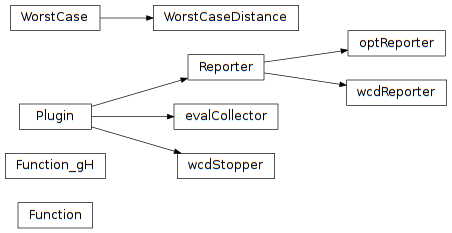# 6.3. pyopus.design.wcd — Worst case distance computation¶Worst case distance analysis (PyOPUS subsystem name: WCD)

Computes the worst case distances of the circuit’s performances. Statistical parameters are assumed to be independent with zero mean and standard deviation equal to 1.

class pyopus.design.wcd.WorstCaseDistance(heads, analyses, measures, statParamDesc, opParamDesc, fixedParams={}, variables={}, debug=0, linearWc=True, alternating=True, maxPass=20, wcStepTol=0.01, stepTol=0.01, constrTol=0.01, angleTol=15, stepScaling=4, perturbationScaling=64, screenThreshold=0.01, maximalStopperStep=0.5, evaluatorOptions={}, sensOptions={}, screenOptions={}, opOptimizerOptions={}, optimizerOptions={}, spawnerLevel=1)

See the PerformanceEvaluator class for details on heads, analyses, measures, and variables.

See the WorstCase class for the explanation of statParamDesc, opParamDesc, fixedParams, maxPass, wcStepTol, stepTol, constrTol, angleTol, stepScaling, perturbationScaling, screenThreshold, maximalStopperStep, evaluatorOptions, sensOptions, screenOptions, opOptimizarOptions, optimizerOptions, and spawnerLevel options.

Setting debug to a value greater than 0 turns on debug messages. The verbosity is proportional to the specified number.

If linearWc is set to True the initial point in the space of the statistical parameters is computed using linear worst case distance analysis.

This is a callable object with an optional argument specifying which worst case distances to compute. If given, the argument must be a list of entries. Every entry is

• a tuple of the form (name,type), where name is the measure name and type is lower or upper
• a string specifying the measure name. In this case the type of comuted worst case distance is given by the presence of the lower and the upper entries in the measure’s description.

If no argument is specified, all worst case distances corresponding to lower/upper bounds of all measures are computed.

The results are stored in the results member. They are represented as a list of dictionaries with the following members:

• name - name of the performance measure
• type - lower or upper
• nominal - nominal performance
• nominal_wcop - performance at nominal statistical parameters and initial worst case operating parameters
• linwc - performance at the linearized worst case distance point
• wc - performance at the worst case distance point
• scr_stat - list of statistical parameters (screened)
• scr_op - list of op parameters (screened)
• lindist - linearized worst case distance
• dist - worst case distance
• op - operating parameter values at the worst case distance point
• stat - statistical parameter values at the worst case distance point
• evals - number of evaluations
• status - OK, FAILED, OUTSIDE+, OUTSIDE-, SENS+, or SENS-

Status FAILED means that the algorithm failed to converge in maxPass passes.

Status OUTSIDE+ means that the algorithm faield to find a point satisfying the WCD search constraint for positive WCD. This means that the WCD is a large positive value.

Status OUTSIDE- means that the algorithm faield to find a point satisfying the WCD search constraint for negative WCD. This means that the WCD is a large negative value.

SENS+ and SENS- are similar to OUTSIDE+ and OUTSIDE-, except that they occur when zero sensitivity to statistical parameters is detected.

Objects of this type store the number of analyses performed during the last call to the object in a dictionary stored in the analysisCount member.

The return value of a call to an object of this class is a tuple holding the results structure and the analysis count dictionary.

formatResults(results=None, nMeasureName=10, nResult=14, nPrec=5, nEvalPrec=4, details=False)

Formats the results as a string.

nMeasureName specifies the formatting width for the performance measure name.

nResult and nPrec specify the formatting width and the number of significant digits for the performance measure values.

nEvalPrec is the number of spaces reserved for the evaluations count.

If details is True the nominal performance, the performance the worst case operating parameters and the nominal statistical parameters, and the linear worst case distance are also formatted.

#### Previous topic

6.2. pyopus.design.wc — Worst case performance computation

#### Next topic

6.4. pyopus.design.mc — Monte Carlo analysis